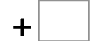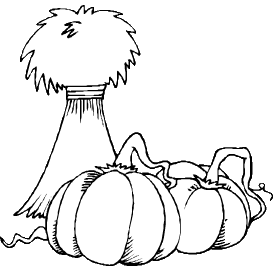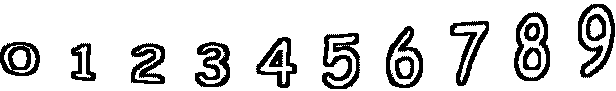88= 169   54= 10326= 49   85= 133   44= 79   57= 98 90= 118   63= 158   97= 153   34= 69